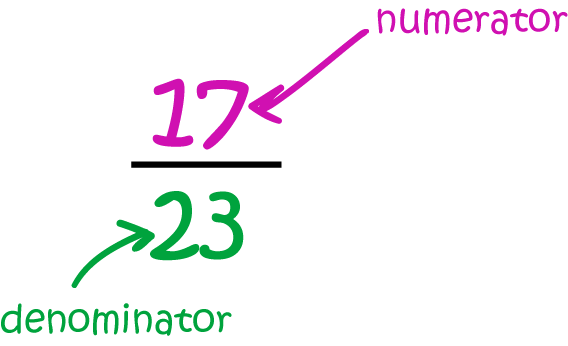# Definition of NumeratorA numerator is the numeral up the top of a fraction.

It tells you how many parts of an object or number there are.

The numeral down the bottom of a fraction is called its denominator. It tells you how many equal parts an object or number has been divided into.

### Description

The aim of this dictionary is to provide definitions to common mathematical terms. Students learn a new math skill every week at school, sometimes just before they start a new skill, if they want to look at what a specific term means, this is where this dictionary will become handy and a go-to guide for a student.

### Audience

Year 1 to Year 12 students

### Learning Objectives

Learn common math terms starting with letter N

Author: Subject Coach
You must be logged in as Student to ask a Question.